# Variations with repetition

Find out how many different ways you can choose k items from n items set. With/without repetition, with/without order.

(n)
(k)

3521614606208

# A bit of theory - foundation of combinatorics

## Variations

A variation of the k-th class of n elements is an ordered k-element group formed from a set of n elements. The elements are not repeated and depend on the order of the group's elements (therefore arranged).

The number of variations can be easily calculated using the combinatorial rule of product. For example, if we have the set n = 5 numbers 1,2,3,4,5 and we have to make third-class variations, their V3 (5) = 5 * 4 * 3 = 60.
${V}_{k}\left(n\right)=n\left(n-1\right)\left(n-2\right)\mathrm{.}\mathrm{.}\mathrm{.}\left(n-k+1\right)=\frac{n!}{\left(n-k\right)!}$
n! we call the factorial of the number n, which is the product of the first n natural numbers. The notation with the factorial is only clearer, equivalent. For calculations, it is fully sufficient to use the procedure resulting from the combinatorial rule of product.

## Permutations

The permutation is a synonymous name for a variation of the nth class of n-elements. It is thus any n-element ordered group formed of n-elements. The elements are not repeated and depend on the order of the elements in the group.
$P\left(n\right)=n\left(n-1\right)\left(n-2\right)\mathrm{.}\mathrm{.}\mathrm{.}1=n!$
A typical example is: We have 4 books, and in how many ways can we arrange them side by side on a shelf?

## Variations with repetition

A variation of the k-th class of n elements is an ordered k-element group formed of a set of n elements, wherein the elements can be repeated and depends on their order. A typical example is the formation of numbers from the numbers 2,3,4,5, and finding their number. We calculate their number according to the combinatorial rule of the product:
${V}_{k}^{\mathrm{\prime }}\left(n\right)=n\cdot n\cdot n\cdot n\mathrm{.}\mathrm{.}\mathrm{.}n={n}^{k}$

## Permutations with repeat

A repeating permutation is an arranged k-element group of n-elements, with some elements repeating in a group. Repeating some (or all in a group) reduces the number of such repeating permutations.
${P}_{{k}_{1}{k}_{2}{k}_{3}\mathrm{.}\mathrm{.}\mathrm{.}{k}_{m}}^{\mathrm{\prime }}\left(n\right)=\frac{n!}{{k}_{1}!{k}_{2}!{k}_{3}!\mathrm{.}\mathrm{.}\mathrm{.}{k}_{m}!}$
A typical example is to find out how many seven-digit numbers formed from the numbers 2,2,2, 6,6,6,6.

## Combinations

A combination of a k-th class of n elements is an unordered k-element group formed from a set of n elements. The elements are not repeated, and it does not matter the order of the group's elements. In mathematics, disordered groups are called sets and subsets. Their number is a combination number and is calculated as follows:
${C}_{k}\left(n\right)=\left(\genfrac{}{}{0px}{}{n}{k}\right)=\frac{n!}{k!\left(n-k\right)!}$
A typical example of combinations is that we have 15 students and we have to choose three. How many will there be?

## Combinations with repeat

Here we select k element groups from n elements, regardless of the order, and the elements can be repeated. k is logically greater than n (otherwise, we would get ordinary combinations). Their count is:
${C}_{k}^{\mathrm{\prime }}\left(n\right)=\left(\genfrac{}{}{0px}{}{n+k-1}{k}\right)=\frac{\left(n+k-1\right)!}{k!\left(n-1\right)!}$
Explanation of the formula - the number of combinations with repetition is equal to the number of locations of n − 1 separators on n-1 + k places. A typical example is: we go to the store to buy 6 chocolates. They offer only 3 species. How many options do we have? k = 6, n = 3.

## Foundation of combinatorics in word problemsOn the menu are 12 kinds of meal. How many ways can we choose four different meals into the daily menu?
• Tokens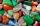In the non-transparent bags are red, white, yellow, blue tokens. We 3times pull one tokens and again returned it, write down all possibilities.
• First manWhat is the likelihood of a random event where are five men and seven women first will leave the man?
• MedalsIn how many ways can be divided gold, silver, and bronze medal among 21 contestants?
• Olympics metalsIn how many ways can win six athletes medal positions in the Olympics? Metal color matters.
• Three shooters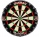Three shooters shoot, each one time, on the same target. The first hit the target with a probability of 0.7; second with a probability of 0.8 and a third with a probability of 0.9. What is the probability to hit the target: a) just once b) at least once c
• Win in raffle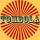The raffle tickets were sold 200, 5 of which were winning. What is the probability that Peter, who bought one ticket will win?
• CombinationsHow many different combinations of two-digit number divisible by 4 arises from the digits 3, 5 and 7?
• Peak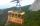Uphill leads 2 paths and 1 lift. a) How many options back and forth are there? b) How many options to get there and back by not same path are there? c) How many options back and forth are there that we go at least once a lift?
• Telephone numbers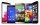How many 7-digit telephone numbers can we put together so that each number consists of different digits?
• How many 2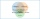How many three-lettered words can be formed from letters A B C D E G H if repeats are: a) not allowed b) allowed.
• Find the 2Find the term independent of x in the expansion of (4x3+1/2x)8
• Football league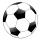In the 5th football league is 10 teams. How many ways can be filled first, second and third place?
• Seven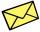Seven friends agree to send everyone a holiday card. How many postcards were sent?
• Tournament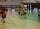Determine how many ways can be chosen two representatives from 34 students to school tournament.
• Five-digitFind all five-digit numbers that can be created from numbers 12345 so that the numbers are not repeated and then numbers with repeated digits. Give the calculation.